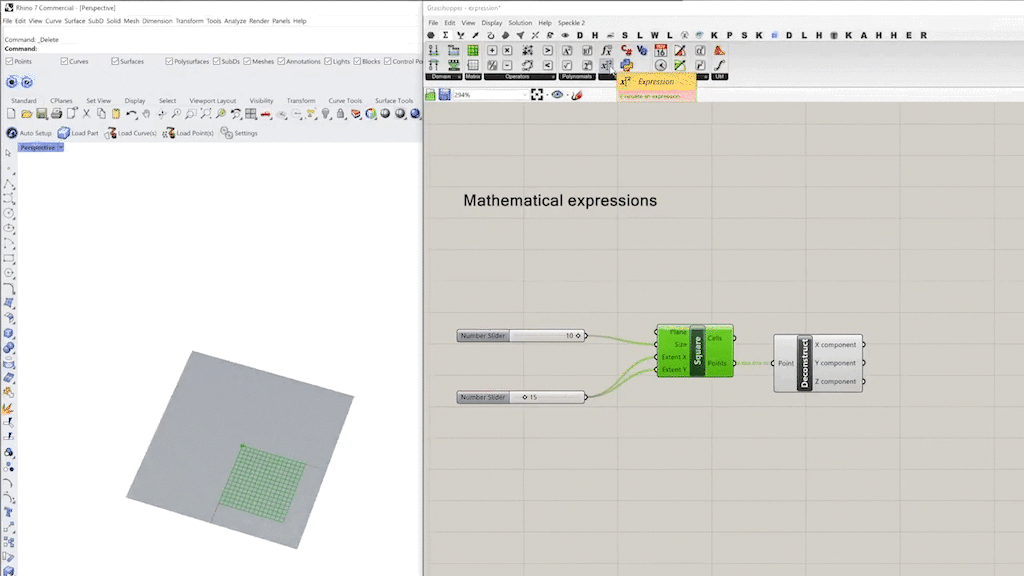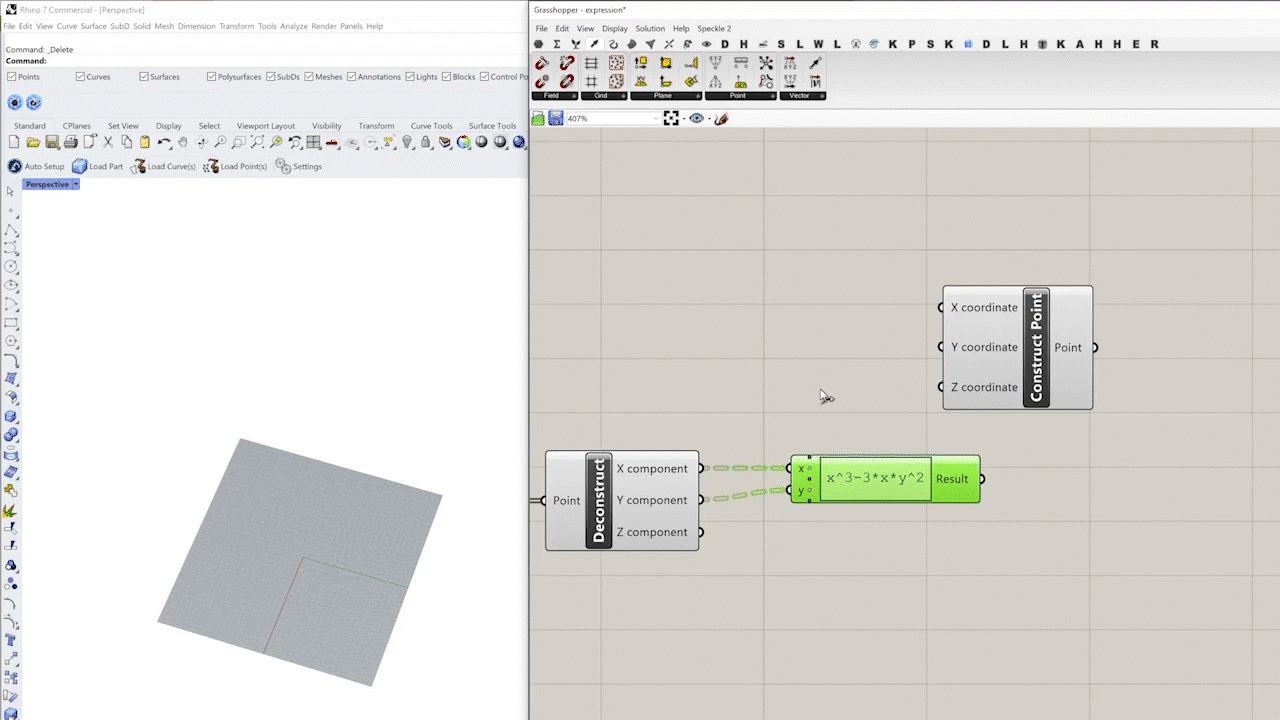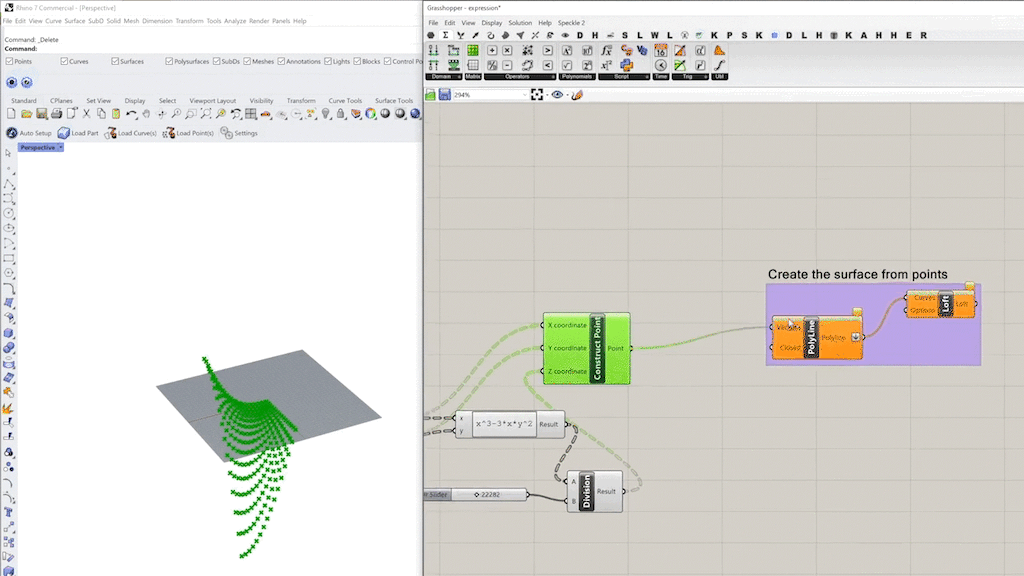top of page
Search

# 018 – Mathematical expressions in Grasshopper

Updated: May 16

## Mathematical Expressions

We can solve mathematical formulas and output numerical data by using an expression component. In this example, we will create a saddle surface which is a mathematical surface using the expression component.

With the expression component, you can perform complex calculations, manipulate variables, and automate repetitive tasks. This can be particularly useful when working with large amounts of data or when you need to perform iterative calculations. One of the main benefits of using mathematical expressions in Grasshopper is that they allow you to easily perform complex calculations and transformations on your data. This can help you to quickly test and explore different design scenarios, saving time and improving the overall efficiency of your design process.

## Steps:

Step 1: First you will need x,y,z coordinates to create a surface. We can use the 'grid' component to create a grid of points.Step 2: From the grid we use the 'deconstruct points' component to extract the

x,y,z coordinates.Step 3: Now we need to use the 'expression' component. The simple Cartesian equation for the saddle surface is z=x(x^2-3y^2). But for simplicity we will write it as z=x^3-3*x*y^2.Note: The input variables should be the same as in the formula. In this case, x and y.

Step 4: Now using the new z variable from the formula, we can create the new point set with the 'construct point' component.Note: Since we are dealing with a large number derived from the formula, we need to use the 'divide' component to make z smaller.

And this is the result: